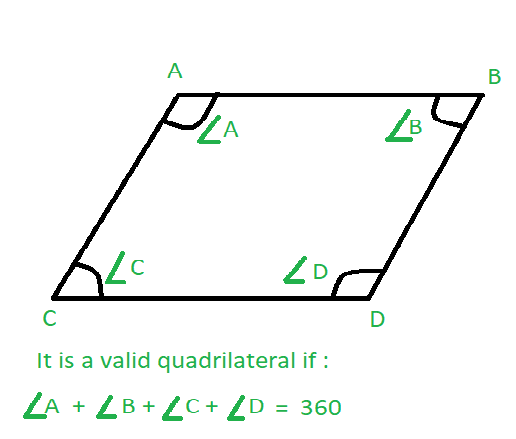# Check whether Quadrilateral is valid or not if angles are given

Given four integers A, B, C and D which represents the four angles of a Quadrilateral in degrees. The task is to check whether the given quadrilateral is valid or not.Examples:

Input: A = 80, B = 70, C = 100, D=110
Output: Valid
Input: A = 70, B = 80, C = 130, D=60
Output: Invalid

## Recommended: Please try your approach on {IDE} first, before moving on to the solution.

Approach:
A Quadrilateral is valid if the sum of the four angles is equal to 360 degrees.

Below is the implementation of the above approach:

## C++

 `// C++ program to check if a given  ` `// quadilateral is valid or not ` `#include ` `using` `namespace` `std; ` ` `  `// Function to check if a given  ` `// quadilateral is valid or not ` `bool` `Valid(``int` `a, ``int` `b, ``int` `c, ``int` `d) ` `{ ` `    ``// Check condition ` `    ``if` `(a + b + c + d == 360) ` `        ``return` `true``; ` `     `  `    ``return` `false``; ` `} ` ` `  `// Driver code ` `int` `main() ` `{ ` `    ``int` `a = 80, b = 70, c = 100, d = 110; ` ` `  `    ``if` `(Valid(a, b, c, d)) ` `        ``cout << ``"Valid quadilateral"``; ` `    ``else` `        ``cout << ``"Invalid quadilateral"``; ` `         `  `    ``return` `0; ` `} `

## Java

 `// Java program to check if a given  ` `// quadilateral is valid or not  ` `class` `GFG ` `{ ` `     `  `// Function to check if a given  ` `// quadilateral is valid or not  ` `public` `static` `int` `Valid(``int` `a, ``int` `b,  ` `                        ``int` `c, ``int` `d)  ` `{  ` `    ``// Check condition  ` `    ``if` `(a + b + c + d == ``360``)  ` `        ``return` `1``;  ` `     `  `    ``return` `0``;  ` `}  ` ` `  `// Driver code  ` `public` `static` `void` `main (String[] args)  ` `{ ` `    ``int` `a = ``80``, b = ``70``, c = ``100``, d = ``110``;  ` `     `  `    ``if` `(Valid(a, b, c, d) == ``1``)  ` `        ``System.out.println(``"Valid quadilateral"``);  ` `    ``else` `        ``System.out.println(``"Invalid quadilateral"``);  ` `}  ` `} ` ` `  `// This code is contributed ` `// by apurva_sharma244 `

## Python3

 `# Python program to check if a given  ` `# quadilateral is valid or not ` ` `  `# Function to check if a given  ` `# quadilateral is valid or not ` `def` `Valid(a, b, c, d): ` ` `  `    ``# Check condition ` `    ``if` `(a ``+` `b ``+` `c ``+` `d ``=``=` `360``): ` `        ``return` `True``; ` `     `  `    ``return` `False``; ` ` `  ` `  `# Driver code ` `a ``=` `80``; b ``=` `70``; c ``=` `100``; d ``=` `110``; ` ` `  `if` `(Valid(a, b, c, d)): ` `    ``print``(``"Valid quadilateral"``); ` `else``: ` `    ``print``(``"Invalid quadilateral"``); ` ` `  `# This code is contributed by Rajput-Ji `

## C#

 `// C# program to check if a given  ` `// quadilateral is valid or not   ` `class` `GFG  ` `{ ` ` `  `// Function to check if a given  ` `// quadilateral is valid or not  ` `static` `bool` `Valid(``int` `a, ``int` `b,  ` `                  ``int` `c, ``int` `d)  ` `{  ` `    ``// Check condition  ` `    ``if` `(a + b + c + d == 360)  ` `        ``return` `true``;  ` `     `  `    ``return` `false``;  ` `}  ` ` `  `// Driver code  ` `public` `static` `void` `Main() ` `{ ` `    ``int` `a = 80, b = 70, c = 100, d = 110;  ` `     `  `    ``if` `(Valid(a, b, c, d))  ` `        ``Console.WriteLine(``"Valid quadilateral"``);  ` `    ``else` `        ``Console.WriteLine(``"Invalid quadilateral"``);  ` `}  ` `} ` ` `  `// This code is contributed by nidhiva `

## PHP

 `  `

Output:

```Valid quadilateral
```

GeeksforGeeks has prepared a complete interview preparation course with premium videos, theory, practice problems, TA support and many more features. Please refer Placement 100 for details

My Personal Notes arrow_drop_upCheck out this Author's contributed articles.

If you like GeeksforGeeks and would like to contribute, you can also write an article using contribute.geeksforgeeks.org or mail your article to contribute@geeksforgeeks.org. See your article appearing on the GeeksforGeeks main page and help other Geeks.

Please Improve this article if you find anything incorrect by clicking on the "Improve Article" button below.